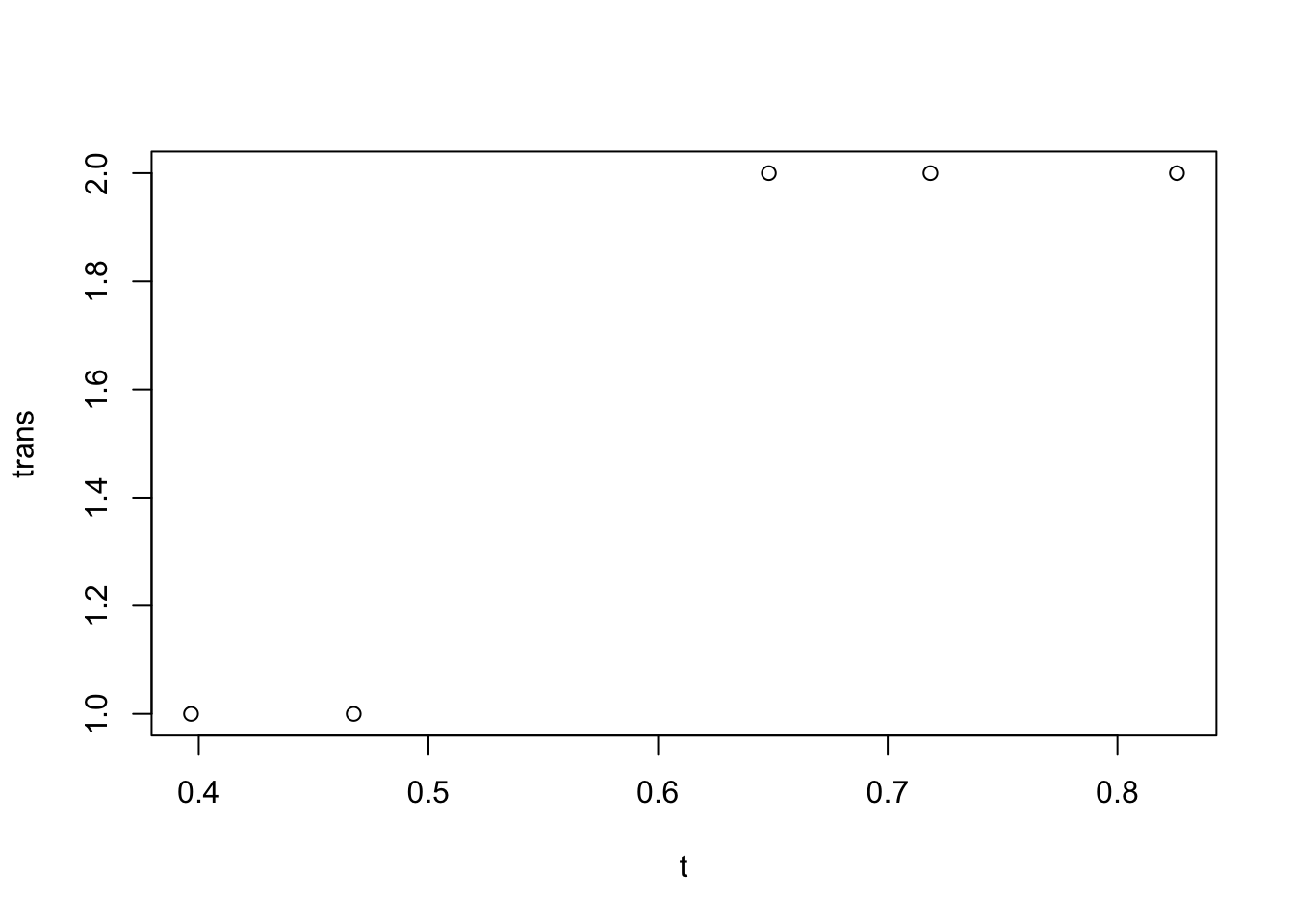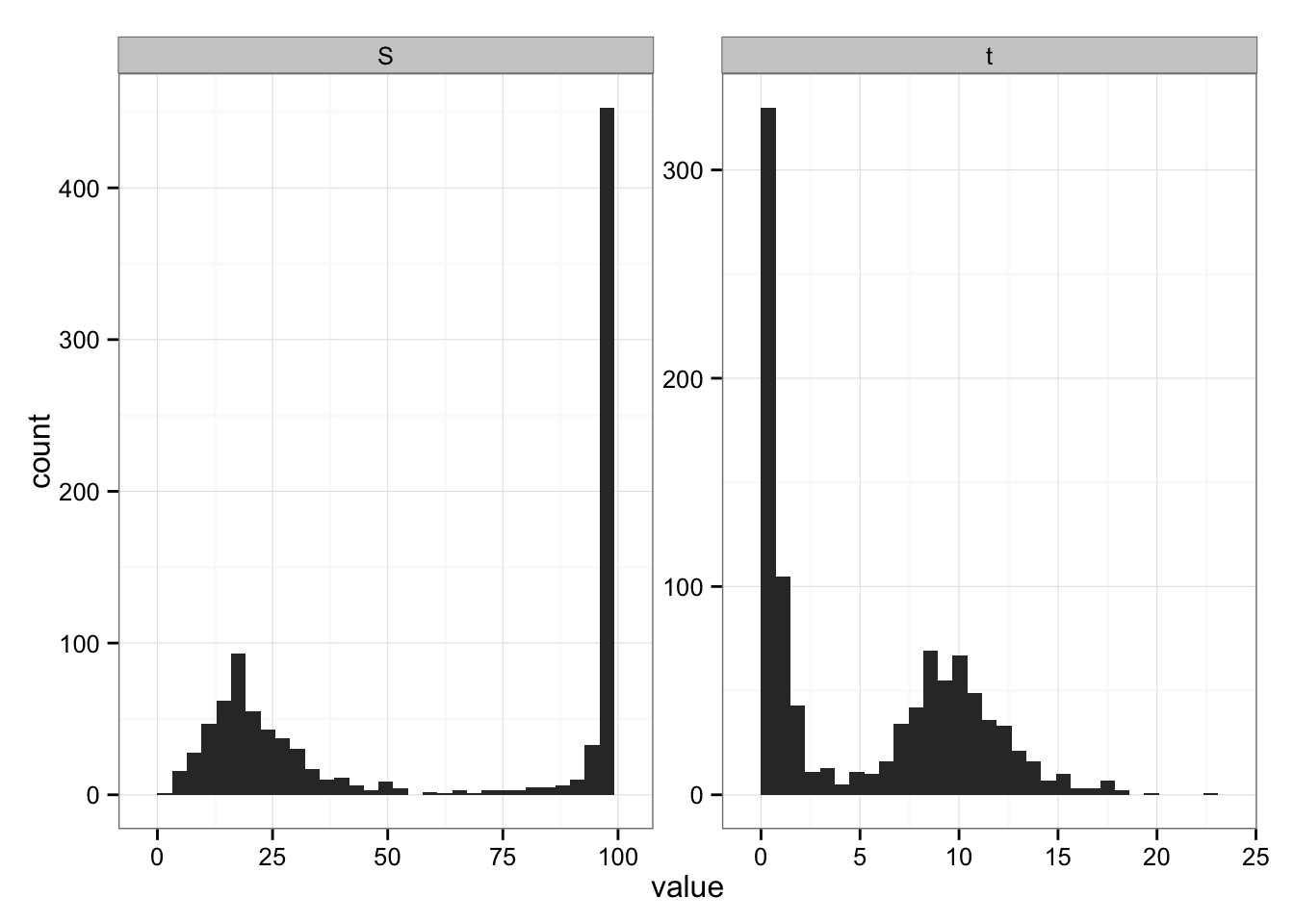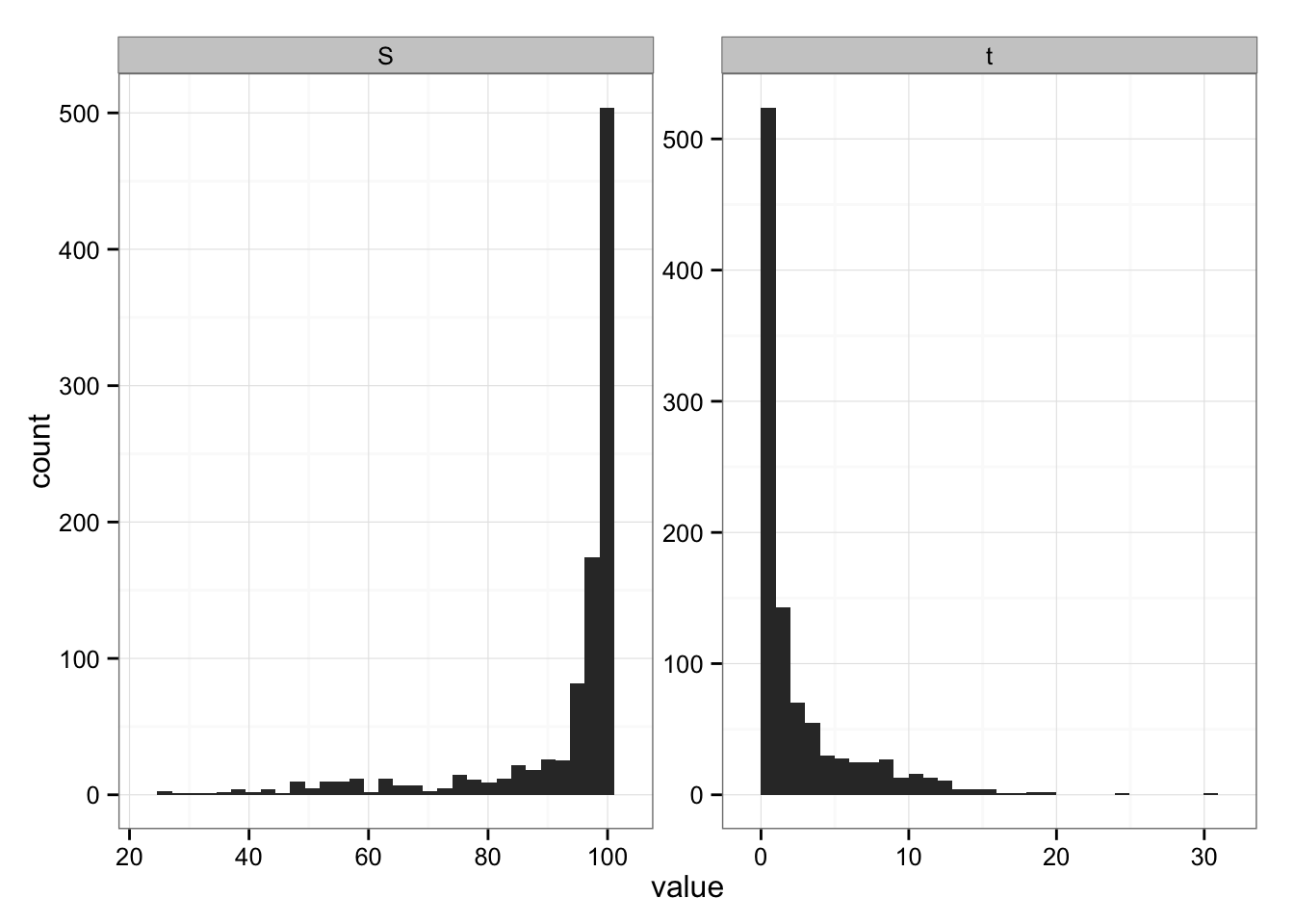I wanted to run some continuous-time, stochastic simulations of the basic SIR model. What’s here is stuff that I and others have probably written hundreds of versions of over the years, but I thought it might be interesting to others as a worked example. Here I’m using the Gillespie algorithm, the simplest brute-force method for discrete-state, continuous-time stochastic simulation. The Gillespie algorithm is implemented in many other places, e.g. the GillespieSSA package for R, and in Darren Wilkinson’s smfsb (“Stochastic Modeling for Systems Biology”) package, along with other more sophisticated/efficient variations such as tau-leap algorithms, but this is the quick and dirty version.

Packages:

library("plyr")     ## for rdply()
library("reshape2") ## for melt()
library("emdbook")  ## for lambertW()
library("ggplot2"); theme_set(theme_bw())

Functions for computing the event rates and the transitions to be executed when the events occur:

ratefun <- function(x,p) {
with(as.list(c(x,p)),{
c(inf=beta*S*I/N,  ## scale inf by pop size
recover=gamma*I)
})
}
transfun <- function(x,w) {
switch(w,
x + c(-1,1),   ## infection: S-1, I+1
x + c(0,-1))   ## removal: I-1
}          

A wrapper function to run the simulation with specified parameters/starting values and return either the ending state or a matrix of event times and types:

run <- function(p=c(beta=2,gamma=1,N=100),
I0=1,
itmax=1e5,
ret=c("final","all")) {
ret <- match.arg(ret)
if (ret=="all") {
rmat <- matrix(NA,nrow=itmax,ncol=2,
dimnames=list(NULL,c("t","trans")))
}
x <- c(S=unname(p["N"])-I0,I=I0)
it <- 1
t <- 0
trans <- c(0,0)
while (x["I"]>0 & it<itmax) {
r <- ratefun(x,p)
t <- t+rexp(1,rate=sum(r))
w <- sample(length(r),size=1,prob=r)
x <- transfun(x,w)
if (ret=="all") rmat[it,] <- c(t,w)
it <- it+1
}
if (ret=="all") return(rmat[!is.na(rmat[,1]),])
return(c(S=unname(x["S"]),t=t,it=it))
}

In this particular case the epidemic dies out early, after 2 infections and 3 recoveries (we started with a single infected individual …)

set.seed(101)
ex0 <- run(ret="all")
plot(trans~t,data=ex0)## can use .progress="text" if running interactively
ex1 <- rdply(1e3,run())
ex2 <- rdply(1e3,run(p=c(beta=1.1,gamma=1,N=100)))

Some handy functions:

## convenience function: we only want to keep final
## fraction unaffected, time to extinction ...
mm <- function(x) {
melt(x[c("S","t")],id.var=character(0))
}
## analytic computation of expected final size
## from ?lambertW
finalsize <- function(R0) {
1+1/R0*lambertW(-R0*exp(-R0))
}

Results with $${\cal R}_0=2$$ (default) and $${\cal R}_0=1.1$$:

(g0 <- ggplot(mm(ex1),aes(x=value))+geom_histogram()+
facet_wrap(~variable,scale="free"))finalsize(2)  ## check final size (should add to plot)
##  0.7968121
g0 %+% mm(ex2)